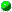`All Packages  Class Hierarchy  This Package  Previous  Next  Index  `

# Class Supporting.Random

```java.lang.Object
|
+----Supporting.Random
```

public class Random
extends Object
Random number class, using a 31-bit linear congruential generator. Note that java.util contains a class Random, so watch out for name conflicts.

##Random()
Construct this Random object with initial state obtained from system clock.Random(int)
Construct this Random object with specified initial state.

##negExp(double)
Return an double using a negative exponential distribution, and change the internal state.permute(Object[])
Randomly rearrange an array.poisson(double)
Return an int using a Poisson distribution, and change the internal state.randomInt()
Return a pseudorandom int, and change the internal state.randomInt(int, int)
Return an int in the closed range [low,high], and change the internal state.randomReal()
Return a double in the open range (0,1), and change the internal state.

##Random
`public Random()`
Construct this Random object with initial state obtained from system clock.Random
`public Random(int initialValue)`
Construct this Random object with specified initial state.

Parameters:
initialValue - the initial state.

##randomInt
`public int randomInt()`
Return a pseudorandom int, and change the internal state.

Returns:
the pseudorandom int.randomReal
`public double randomReal()`
Return a double in the open range (0,1), and change the internal state.

Returns:
the pseudorandom double.randomInt
```public int randomInt(int low,
int high)```
Return an int in the closed range [low,high], and change the internal state.

Parameters:
low - the minimum value returned.
high - the maximum value returned.
Returns:
the pseudorandom int.poisson
`public int poisson(double expectedValue)`
Return an int using a Poisson distribution, and change the internal state.

Parameters:
expectedValue - the mean of the distribution.
Returns:
the pseudorandom int.negExp
`public double negExp(double expectedValue)`
Return an double using a negative exponential distribution, and change the internal state.

Parameters:
expectedValue - the mean of the distribution.
Returns:
the pseudorandom double.permute
`public static final void permute(Object[] a)`
Randomly rearrange an array. The random numbers used depend on the time and day.

Parameters:
a - the array.

`All Packages  Class Hierarchy  This Package  Previous  Next  Index  `